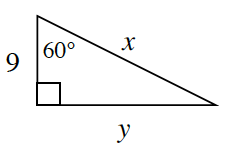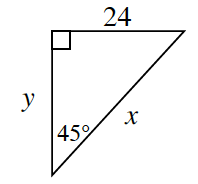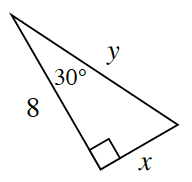Home > CCG > Chapter Ch7 > Lesson 7.1.1 > Problem7-10

7-10.

Without using your calculator, find the exact values of $x$ and $y$ in each diagram below.

All three triangles are special right triangles. Refer to the Math Note from Lesson 5.3.1 for shortcuts to find the lengths of sides of special right triangles.

1.$x=18$$y=9\sqrt{3}$

1.$x=24\sqrt{2}$$y=24$

1.Refer to parts (a) and (b).